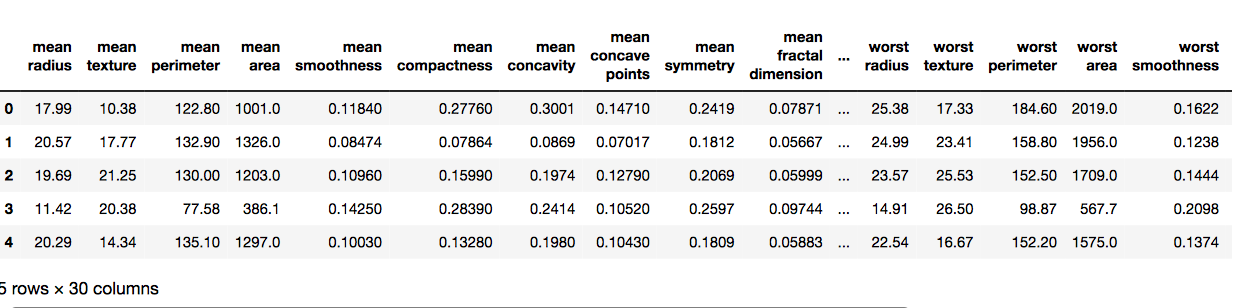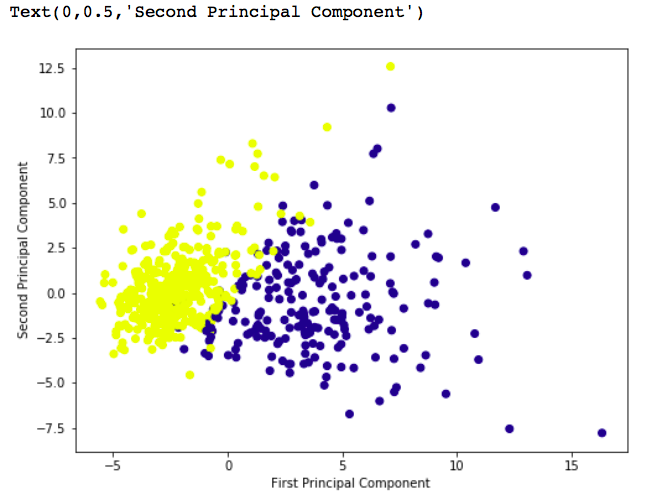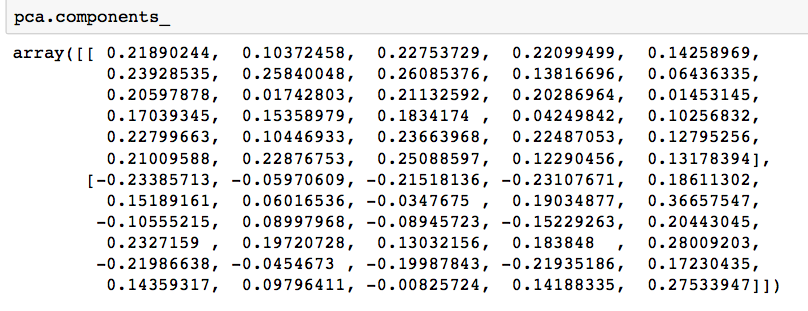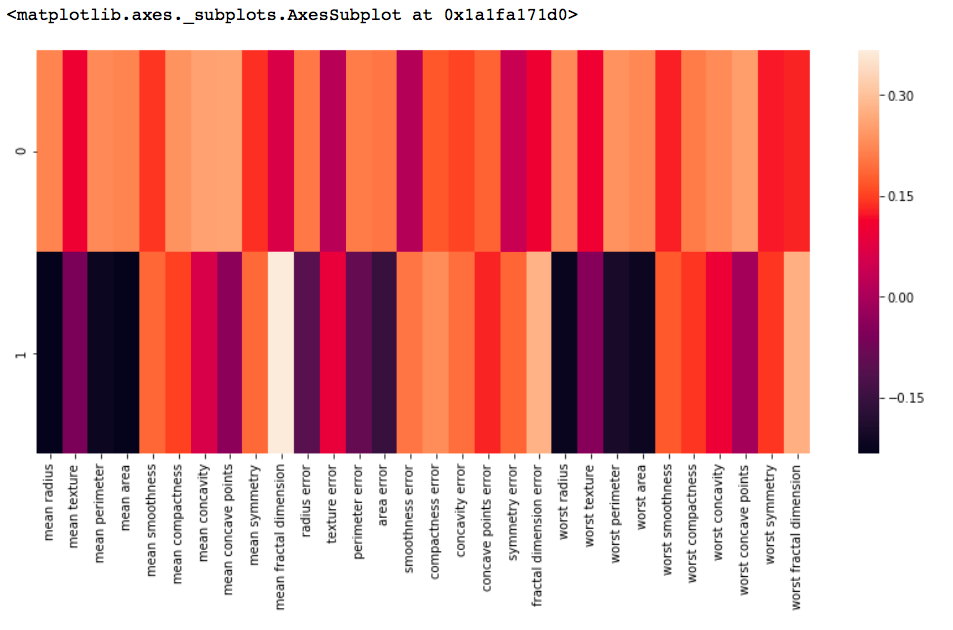Open in App
Not now

## Related Articles

• Write an Interview Experience
• Machine Learning

# ML | Principal Component Analysis(PCA)

• Difficulty Level : Medium
• Last Updated : 13 Mar, 2023

This method was introduced by Karl Pearson. It works on a condition that while the data in a higher dimensional space is mapped to data in a lower dimension space, the variance of the data in the lower dimensional space should be maximum.

Principal Component Analysis (PCA) is a statistical technique used to reduce the dimensionality of a large dataset. It is a commonly used method in machine learning, data science, and other fields that deal with large datasets.

1. PCA works by identifying patterns in the data and then creating new variables that capture as much of the variation in the data as possible. These new variables, known as principal components, are linear combinations of the original variables in the dataset.
2. The first principal component captures the most variation in the data, the second captures the second most, and so on. The number of principal components created is equal to the number of original variables in the dataset.
3. PCA can be used for a variety of purposes, including data visualization, feature selection, and data compression. In data visualization, PCA can be used to plot high-dimensional data in two or three dimensions, making it easier to interpret. In feature selection, PCA can be used to identify the most important variables in a dataset. In data compression, PCA can be used to reduce the size of a dataset without losing important information.

Overall, PCA is a powerful tool for data analysis and can help to simplify complex datasets, making them easier to understand and work with.

Principal Component Analysis (PCA) is to reduce the dimensionality of a data set by finding a new set of variables, smaller than the original set of variables, retains most of the sample’s information and useful for the compression and classification of data.

In PCA, it is assumed that the information is carried in the variance of the features, that is, the higher the variation in a feature, the more information that feature carries.

Hence, PCA employs a linear transformation that is based on preserving the most variance in the data using the least number of dimensions. It involves the following steps:

1. Construct the covariance matrix of the data.

2. Compute the eigenvectors of this matrix.

3. Eigenvectors corresponding to the largest eigen values are used to reconstruct a large fraction of variance of the original data.

The data instances are projected onto a lower dimensional space where the new features best represent the entire data in the least squares sense.

It can be shown that the optimal approximation, in the least square error sense, of a d-dimensional random vector x2< d by a linear combination of independent vectors is obtained by projecting the vector x onto the eigenvectors e1 corresponding to the largest eigen values l1 of the covariance matrix (or the scatter matrix) of the data from which x is drawn.

The eigenvectors of the covariance matrix of the data are referred to as principal axes of the data, and the projection of the data instances on to these principal axes are called the principal components. Dimensionality reduction is then obtained by only retaining those axes (dimensions) that account for most of the variance, and discarding all others.

Principal Component Analysis (PCA) is a statistical procedure that uses an orthogonal transformation that converts a set of correlated variables to a set of uncorrelated variables.PCA is the most widely used tool in exploratory data analysis and in machine learning for predictive models. Moreover, PCA is an unsupervised statistical technique used to examine the interrelations among a set of variables. It is also known as a general factor analysis where regression determines a line of best fit. Module Needed:

## Python3

 `import` `pandas as pd``import` `numpy as np``import` `matplotlib.pyplot as plt``import` `seaborn as sns``%``matplotlib inline`

Code #1:

## Python3

 `# Here we are using inbuilt dataset of scikit learn``from` `sklearn.datasets ``import` `load_breast_cancer` `# instantiating``cancer ``=` `load_breast_cancer()` `# creating dataframe``df ``=` `pd.DataFrame(cancer[``'data'``], columns ``=` `cancer[``'feature_names'``])` `# checking head of dataframe``df.head()`

Output:Code #2:

## Python3

 `# Importing standardscalar module``from` `sklearn.preprocessing ``import` `StandardScaler` `scalar ``=` `StandardScaler()` `# fitting``scalar.fit(df)``scaled_data ``=` `scalar.transform(df)` `# Importing PCA``from` `sklearn.decomposition ``import` `PCA` `# Let's say, components = 2``pca ``=` `PCA(n_components ``=` `2``)``pca.fit(scaled_data)``x_pca ``=` `pca.transform(scaled_data)` `x_pca.shape`

Output:

`569, 2`

## Python3

 `# giving a larger plot``plt.figure(figsize ``=``(``8``, ``6``))` `plt.scatter(x_pca[:, ``0``], x_pca[:, ``1``], c ``=` `cancer[``'target'``], cmap ``=``'plasma'``)` `# labeling x and y axes``plt.xlabel(``'First Principal Component'``)``plt.ylabel(``'Second Principal Component'``)`

Output:## Python3

 `# components``pca.components_`

Output:## Python3

 `df_comp ``=` `pd.DataFrame(pca.components_, columns ``=` `cancer[``'feature_names'``])` `plt.figure(figsize ``=``(``14``, ``6``))` `# plotting heatmap``sns.heatmap(df_comp)`

Output:1. Dimensionality Reduction: PCA is a popular technique used for dimensionality reduction, which is the process of reducing the number of variables in a dataset. By reducing the number of variables, PCA simplifies data analysis, improves performance, and makes it easier to visualize data.
2. Feature Selection: PCA can be used for feature selection, which is the process of selecting the most important variables in a dataset. This is useful in machine learning, where the number of variables can be very large, and it is difficult to identify the most important variables.
3. Data Visualization: PCA can be used for data visualization. By reducing the number of variables, PCA can plot high-dimensional data in two or three dimensions, making it easier to interpret.